# Measuring Length to the Nearest Quarter or Half Inch Online Quiz

#### Complete Python Prime Pack

9 Courses     2 eBooks

#### Artificial Intelligence & Machine Learning Prime Pack

6 Courses     1 eBooks

#### Java Prime Pack

9 Courses     2 eBooks

Following quiz provides Multiple Choice Questions (MCQs) related to Measuring Length to the Nearest Quarter or Half Inch. You will have to read all the given answers and click over the correct answer. If you are not sure about the answer then you can check the answer using Show Answer button. You can use Next Quiz button to check new set of questions in the quiz.Q 1 - Measure the length of the object to the nearest half inch.### Explanation

Step 1:

The reading is nearer to $3\frac{1}{2}$ mark than to 4 mark.

Step 2:

So, the length of the object to nearest half inch is $3\frac{1}{2}$ inches.

Q 2 - Measure the length of the object to the nearest half inch.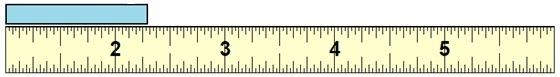### Explanation

Step 1:

The reading is nearer to $2\frac{1}{2}$ mark than to 2 mark.

Step 2:

So, the length of the object to nearest half inch is $2\frac{1}{2}$ inches.

Q 3 - Measure the length of the object to the nearest half inch.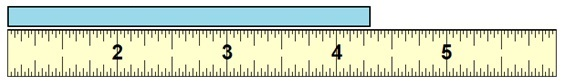### Explanation

Step 1:

The reading is nearer to $4\frac{1}{2}$ mark than to 4 mark.

Step 2:

So, the length of the object to nearest half inch is $4\frac{1}{2}$ inches.

Q 4 - Measure the length of the object to the nearest half inch.### Explanation

Step 1:

The reading is nearer to $2\frac{1}{2}$ mark than to 3 mark.

Step 2:

So, the length of the object to nearest half inch is $2\frac{1}{2}$ inches.

Q 5 - Measure the length of the object to the nearest half inch.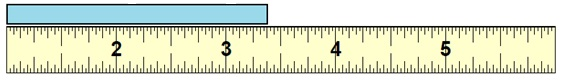### Explanation

Step 1:

The reading is nearer to $3\frac{1}{2}$ mark than to 3 mark.

Step 2:

So, the length of the object to nearest half inch is $3\frac{1}{2}$ inches.

Q 6 - Measure the length of the object to the nearest half inch.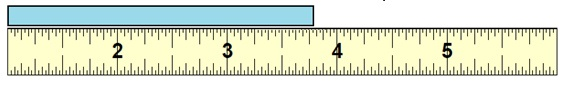### Explanation

Step 1:

The reading is nearer to $3\frac{3}{4}$ mark than to 4 mark.

Step 2:

So, the length of the object to nearest quarter inch is $3\frac{3}{4}$ inches.

Q 7 - Measure the length of the object to the nearest half inch.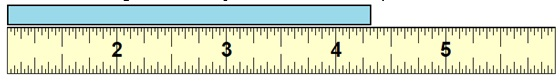### Explanation

Step 1:

The reading is nearer to $4\frac{1}{4}$ mark than to $4\frac{1}{2}$ mark.

Step 2:

So, the length of the object to nearest quarter inch is $4\frac{1}{4}$ inches.

Q 8 - Measure the length of the object to the nearest half inch.### Explanation

Step 1:

The reading is nearer to $4\frac{2}{4}$ mark than to $4\frac{3}{4}$ mark.

Step 2:

So, the length of the object to nearest quarter inch is $4\frac{2}{4}$ inches.

Q 9 - Measure the length of the object to the nearest half inch.### Explanation

Step 1:

The reading is nearer to $3\frac{3}{4}$ mark than to $3\frac{2}{4}$ mark.

Step 2:

So, the length of the object to nearest quarter inch is $3\frac{3}{4}$ inches.

Q 10 - Measure the length of the object to the nearest half inch.### Explanation

Step 1:

The reading is nearer to $5\frac{1}{4}$ mark than to $5\frac{1}{2}$ mark.

Step 2:

So, the length of the object to nearest quarter inch is $5\frac{1}{4}$ inches.

measuring_length_to_nearest_quarter_or_half_inch.htm